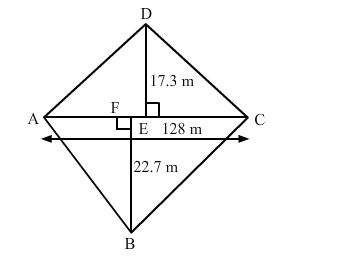# In a four-sides field, the length of the longer diagonal is 128 m.`
Question:

In a four-sides field, the length of the longer diagonal is 128 m. The lengths of perpendiculars from the opposite vertices upon this diagonal are 22.7 m and 17.3 m. Find the area of the field.

Solution:

The field, which is represented as ABCD, is given below.The area of the field is the sum of the areas of triangles ABC and ADC.

Area of the triangle $\mathrm{ABC}=\frac{1}{2}(\mathrm{AC} \times \mathrm{BF})=\frac{1}{2}(128 \times 22.7)=1452.8 \mathrm{~m}^{2}$

Area of the triangle $\mathrm{ADC}=\frac{1}{2}(\mathrm{AC} \times \mathrm{DE})=\frac{1}{2}(128 \times 17.3)=1107.2 \mathrm{~m}^{2}$

Area of the field $=$ Sum of the areas of both the triangles $=1452.8+1107.2=2560 \mathrm{~m}^{2}$